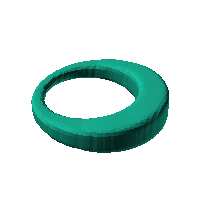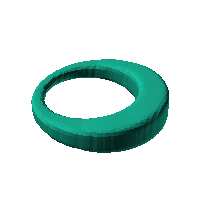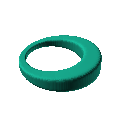Blob Farm# blob27The blob in all its glory:Ring with square cross-section, maybe a good case.

 Click on the snapshot to download the blob's stl file.Octave Code:
```  # name of the blob
project = "blob27";

function w = f(x2,y2,z2,c,r,e)
x  = (x2-c(1))/r(1);
y  = (y2-c(2))/r(2);
z  = (z2-c(3))/r(3);
# function at origin must be <0, and >0 far enough away.  w=0 defines the surface
k = -0.5;
j = -15;
theta = atan2(x,y);
w = -(((sqrt(x.^2+y.^2)-7)./(1+0.5*sin(theta))).^6+(z./(1+0.5*sin(theta))).^6).^k + 0.5;

endfunction;

c_outer = [0,0,0];
r_outer = [10,10,10];

step = 4;  # grid pitch in mm  start with 4mm to see the shape quickly.  Once you have it just right, change to 2mm for printing

source("../octave/func2stl_v01.m");  # do all the calculations

```
GNU Octave